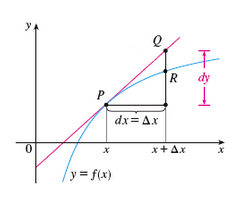## Differentials and Approximation

One advantage of finding equations of tangent lines to points on the graph of a function is that they provide a reasonable approximation to the function near the point in question. That is to say, for an input sufficiently near $x$, the value of the function is approximately the same as the height of the tangent line at that input.

Consider, for a linear function $f(x) = mx + b$ we know that

$$\begin{array}{rcl} f(x + \Delta x) &=& m(x + \Delta x) + b\\ &=& (mx + b) + m \cdot \Delta x\\ &=& f(x) + m \cdot \Delta x \end{array}$$

Let's put these ideas together.

Recall that the slope $m$ of the tangent line to $y=f(x)$ at $x$ (presuming $f$ is differentiable there) is given by $m = f'(x)$.

Then, for sufficiently small $\Delta$ (making the input $x + \Delta x$ sufficiently near $x$), the following should hold for any such function $f$:

$$f(x + \Delta x) \approx f(x) + f'(x) \cdot \Delta x$$

Our requirement above, that $\Delta x$ be sufficiently small, motivates the following definitions:

Let us denote by $dx$ a change in the $x$-coordinate, $\Delta x$, from some $x$ in the domain of a function $f$. We call this $dx$ the differential of $x$.

Let us likewise denote by $dy$ the corresponding change in the $y$ coordinate along the tangent line, calling it the differential of $y$, as shown below.Clearly, given that the slope of the tangent line is $f'(x)$, the following must be true.

$$dy = f'(x) \cdot \Delta x$$

The notations used are suggestive, of course. Note that their quotient must always equal the derivative at $x$:

$$\frac{dy}{dx} = \frac{f'(x) \cdot \Delta x}{\Delta x} = f'(x)$$

With the above in mind, our earlier approximation -- relative to some given function $f$ and some specific value of $x$ -- can now be expressed as

$$f(x + dx) \approx f(x) + dy$$

In terms of the picture shown above, this means that we can approximate the height of the function at some point $R$ with the height of the corresponding point $Q$ on the tangent line. (Granted, these two points don't appear to be at approximately the same height in the picture drawn, but this is because the $\Delta x$ shown was made relatively large so that all of the labels in the picture would fit!)

Consider how we can use this to approximate the value of a function at an input that would normally be difficult to evaluate directly, provided the input is near another input whose output is easily evaluated, as the following examples suggest:

#### Example

Suppose we wish to use differentials to approximate the value of $\sqrt{27.5}$.

Consider the function $f(x) = \sqrt{x}$. If $y=f(x)$, then

$$\begin{array}{rcl} dy &=& f'(x) \cdot dx\\\\ &=& \displaystyle{\frac{1}{3x^{2/3}} dx} \end{array}$$

We know that 27.5 is near the perfect cube $3^3 = 27$, so the related differentials are:

$$dx = 27.5-27 = \frac{1}{2}$$ $$dy = \frac{1}{3 (27)^{2/3}} \cdot \frac{1}{2} = \frac{1}{54}$$

Thus,

$$\begin{array}{rcl} \sqrt{27.5} &=& f(27 + \frac{1}{2})\\ &\approx& f(27) + \frac{1}{54}\\ &=& \sqrt{27} + \frac{1}{54}\\ &=& 3 + \frac{1}{54}\\ &\doteq& 3.018518\ldots \end{array}$$

So how close were we to $\sqrt{27.5}$?

Well, with a calculator we could determine that $\sqrt{27.5} = 3.018405\ldots$

So our approximation was good to three decimal places! Not too shabby, considering the only cube root we took in our calculation was that of 27, a perfect cube!

#### Example

Suppose we wish to use differentials to approximate the value of $\cos 61^{\circ}$.

As a first step, one must recognize that the derivative formulas we know for the various trigonometric functions are appropriate only if the angle is measured in radians. Thus, we first find the radian measure for $61^{\circ}$.

$$61^{\circ} = \frac{61}{180}\pi$$

We'll also need the derivative of $f(x) = \cos x$, which is of course $-\sin x$.

Noting that this radian measure is just $\frac{\pi}{180}$ more than $\frac{\pi}{3}$, we find the corresponding differentials:

$$\textstyle{dx = \frac{61}{180}\pi - \frac{\pi}{3} = \frac{\pi}{180}}$$ $$\begin{array}{rcl} dy &=& f'\left( \frac{\pi}{3} + \frac{\pi}{180} \right)\\ &=& \left( -\sin \left( \frac{\pi}{3} \right) \right) \cdot \frac{\pi}{180}\\ &=& \frac{\sqrt{3}}{2} \cdot \frac{\pi}{180} \end{array}$$

Hence,

$$\begin{array}{rcl} \cos 61^{\circ} &=& \cos(\frac{\pi}{3} + \frac{\pi}{180})\\ &=& \cos(\frac{\pi}{3}) + \frac{\sqrt{3}}{2} \cdot \frac{\pi}{180}\\ &=& \frac{1}{2} + \frac{\sqrt{3}}{2} \cdot \frac{\pi}{180}\\ &\doteq& 0.4849 \end{array}$$

Again, notice how close this is to what $\cos 61^{\circ}$ produces on a calculator (i.e., $0.4848\ldots$).

The impressive part is that we got that close using only our knowledge of the unit circle, addition, multiplication, and a square root!